# Online Linear Regression

On-line linear regression is a subfield of competing with prediction rules in which the strategies in the benchmark class are linear functions. Its basic protocol is:

Players: Forecaster, Reality
Protocol:

Initialize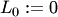FOR:
Reality announces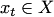Forecaster announces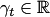Reality announces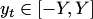END

At the beginning of each step Reality outputs some signal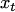from a subset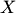of a vector space (typically,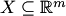for some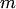). Forecaster's goal is to compete with the linear functions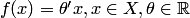. To obtain non-trivial results, it is usually necessary to assume that the outcomes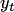are bounded in absolute value by some positive constant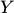, for example as for Aggregating Algorithm Regression.

A theoretical bound for the algorithm competing with linear regressors, and its prediction steps can be described by explicit expressions only in some cases (say, when the initial distribution on experts is chosen to be normal). However in most of the cases the integrals can not be taken, for example in Competitive Online Generalized Linear Regression Under Square Loss. It is possible to deal with this problem by integrating numerically, using Monte-Carlo integration, or more sophisticated MCMC method.

If the classification problem is considered with more than 2 classes, or if each outcome is a probability measure over a finite set of events, Linear Probability Forecasting can be applied to compete with linear functions efficiently.